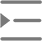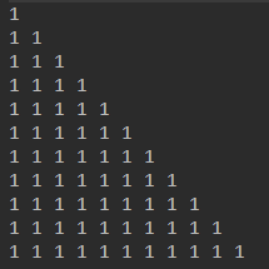# 浅谈Java不规则数组

• 2020-10-26 17:40:40
• 290次 动力节点

Java不规则数组，顾名思义，就是数组本身是“不规则”的。正是因为Java数组的特征，所以在Java数组中可以创建一个“不规则”数组，即数组的每一行有不同的长度，例如第一行有一列，第二行有两列，依次类推。数组如下图所示：int[ ][ ] rows=new int[ ];

rows =new int;

rows=new int;

rows=new int;

public class Main {

public static void main(String args[]) {

final int MAX = 10;

int [][] arrs = new int[MAX + 1][]; //分配一个具有所含行数的数组

for (int n = 0; n <= MAX; n++) //分配每一行

arrs[n] = new int[n + 1];

//给数组赋值

for (int n = 0; n < arrs.length; n++)

for (int k = 0; k < arrs[n].length; k++){

int lotteryArrs = 1;

arrs[n][k] = lotteryArrs;

}

//遍历数组

for (int[] row : arrs){

for (int arr : row)

System.out.print(arr + " ");

System.out.println();

}

}

}

package SE;

import java.util.Random;

public static void main(String[] args)

static void irregular(){

int arrays[][];

arrays = new int[];

for(int i=0;i<arrays.length;i++){< p="">

/*Random r = new Random(47);

int h = r.nextInt(13);*/

int h = (int)(Math.random()*16);

arrays[i] = new int[h];

for(int j=0;j<h;j++){< p="">

int w = (int)(Math.random()*100);

arrays[i][j] = w;

}

}

//遍历输出

for(int i=0;i<arrays.length;i++){< p="">

for(int j=0;j<arrays[i].length;j++){< p="">

System.out.print(arrays[i][j]+" ");

}

System.out.println();

}

}

static void out1(String[] ss){

for (String string : ss) {

System.out.print(string);

}

System.out.println();

}

static void out2(String... ss){

for (String str : ss) {

System.out.print(str);

}

System.out.println();

}

}